# Center of a data set: mean, median, mode#### Everything You Need in One Place

Homework problems? Exam preparation? Trying to grasp a concept or just brushing up the basics? Our extensive help & practice library have got you covered.#### Learn and Practice With Ease

Our proven video lessons ease you through problems quickly, and you get tonnes of friendly practice on questions that trip students up on tests and finals.#### Instant and Unlimited Help

Our personalized learning platform enables you to instantly find the exact walkthrough to your specific type of question. Activate unlimited help now!##### Intros
###### Lessons
1. Overview: Mean, Median, and Mode
2. What is weighted mean?
##### Examples
###### Lessons
1. Determine the mean of each set of data:
a) {8, 5, 2, 12, 3}
b) {7, 5, 3, 8, 3, 4}
1. Determine the median of each set of data:
a) {8, 5, 2, 12, 3}
b) {7, 5, 3, 8, 3, 4}
1. Investigating the Impact of Outliers on the Mean and Median
The heights (in cm) of students in a class are: {156, 152, 148, 159, 150}
a) Determine the mean and the median.
b) A new student with a height of 255 cm joins the class. How does the new data value (outlier)affect the mean and the median?
1. Determine the mode of each set of data:
a) {8, 5, 2, 12, 5}
b) {6, 6, 6, 7, 7, 8, 8, 8, 9}
c) {1, 2, 3, 4}
1. Determining the Mean of a Frequency Distribution
Determine the mean for the population of scores in the following frequency table.

 score frequency 4 6 5 3 7 8 9 2

1. Determining the Weighted Mean
Sarah has earned the following averages in her statistics course: homework-98, quizzes-92, tests-84. The overall course grade is comprised of: homework (10%), quizzes (20%), unit tests (40%), and final exam (30%).
a) What is Sarah's grade going into the final exam?
b) What score must Sarah earn on the final exam in order to earn a final grade of 90% for the course?

## Center of data set: Mean, Median, Mode

Now that you know not only about the basic concepts on the classification of data, but also have learnt about the different shapes of distributions that can result through the variety of possible graphic representations of data, it is time that we combine our knowledge on both areas to study the center of a data set.

In statistics, the term center of data usually refers to the scope at which most of the data values have a tendency to go; in other words, when we collect survey or experimentation values that compose a data set, we usually gather data where a certain pattern can be observed, this pattern is the tendency of all the results to go towards a certain side. In a numerical experiment, this tendency is what we would see on the data obtained through measurement, values would go towards the true or real value at which we might not arrive every time due to random or systematic errors in our experimentation; on the other hand, in a statistical survey, these center values would show the cultural and social tendencies producing a similar, or mostly similar result from a population. On this second case, any far away scattered data value result would instantaneously show a sharp difference between most of the population and the personal background of someone who provided such scattered result.

So now you can see the importance of studying the center of data, or the tendencies of the data in order to obtain conclusions in statistical studies; therefore, let us go into the details of the mathematical tools we use to understand data at this level.

#### Mean median mode range definitions

In order to understand what is the mean, median, mode and range of a data set in a more meaningful manner, let us use an example we are already familiar with:

#### Example 1

On our lesson about stem and leaf plots we worked through this example of data set where we collected the ages of the people who attend a yoga class. After asking all 25 of the people in the class, the data obtained about their ages is the following: 21, 16, 34, 33, 57, 18, 44, 41, 63, 72, 54, 44, 39, 30, 45, 45, 61, 18, 29, 27, 55, 48, 59, 66, 70.

We start by ordering the numbers:
16$\;$ 18$\;$ 18$\;$ 21$\;$ 27$\;$ 29$\;$ 30$\;$ 33$\;$ 34$\;$ 39$\;$ 41$\;$ 44$\;$ 44$\;$ 45$\;$ 45$\;$ 48$\;$ 54$\;$ 55$\;$ 57$\;$ 59$\;$ 61$\;$ 63$\;$ 66$\;$ 70$\;$ 72

With this data in mind, let us look at the calculation and definition of mean, median, mode and range:

#### • $\quad$ Mean:

The mean of a data set is the addition of the values divided by the amount of all the values in the data set. Notice that, given this mean definition, this is the same as the arithmetic average of a set of numbers; thus the terms mean and average are usually used as synonyms.
The mean of a data set tries to find the central value of a set by comparing all of the values in the set and producing the average of them; if all of the values in the set were to be equal, the mean of this set would be equal to all of them too.

#### ‣ $\quad$ How to find the mean:

The calculation to find the mean is really simple, just as described in its definition, you only have to obtain the sum of the values in the set and then divide the result by the amount of values in it.
Using the data from our example 1, this is the method on how to find the mean:

Having the data:

$16, 18, 18, 21, 27, 29, 30, 33, 34, 39, 41, 44, 44, 45, 45, 48, 54, 55, 57, 59, 61, 63, 66, 70, 72$

We obtain the mean:

$\overline{X} = \frac{16+18+18+21+27+29+30+33+34+39+41+44+44+45+45+48+54+55+57+59+61+63+66+70+72} {25 }$

$\overline{X} = \frac{1089} {25} = 43.56$

Notice the mean is usually represented as an x with a horizontal line on top.

#### • $\quad$ Median:

What is a median in statistics? When having a set of data values, the median definition tells us that we just need to find the midpoint of the total data set. In other words, if we order the data values from low to high, the definition of median says that the value in the middle of the list is the median.

#### ‣ $\quad$ How to find the median:

And so, how do you find the median? There is a special rule to remember when finding the median of a data set: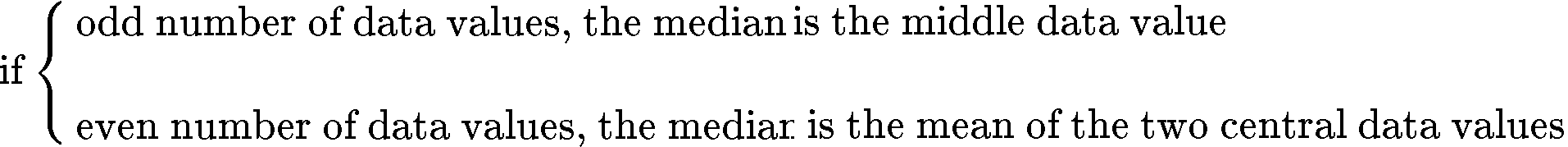Now let us take the list of data from example 1 that is already ordered from lowest to largest:
16$\;$ 18$\;$ 18$\;$ 21$\;$ 27$\;$ 29$\;$ 30$\;$ 33$\;$ 34$\;$ 39$\;$ 41$\;$ 44$\;$ 44$\;$ 45$\;$ 45$\;$ 48$\;$ 54$\;$ 55$\;$ 57$\;$ 59$\;$ 61$\;$ 63$\;$ 66$\;$ 70$\;$ 72

There is really not a method to calculate median values, you just have to identify them. For the data set we have the process is very simple, since we have 25 data values from the list in example 1, then the middle one is the 13th value in the list:

16 $\;$ 18$\;$ 18$\;$ 21$\;$ 27$\;$ 29$\;$ 30$\;$ 33 $\;$ 34 $\;$ 39 $\;$ 41 $\;$ 44$\;$ 44 $\;$ 45 $\;$ 45$\;$ 48 $\;$ 54$\;$ 55$\;$ 57 $\;$ 59$\;$ 61$\;$ 63$\;$ 66$\;$ 70$\;$ 72

And that is the median! Median = 44, that simple.

As you can see, both the mean and median try to achieve the central value of the data set, they try to find the tendency of all the set towards a certain centre. But when comparing mean vs median, which one is best? It all depends of the data set!

If we compare the values of the mean and the median for the data set in example 1, notice that both values are very close to each other, this is because the data set is dispersed nicely towards both sides of the center tendency, thus giving a well defined center of the data. This center can be observed if we graph the data set into a histogram: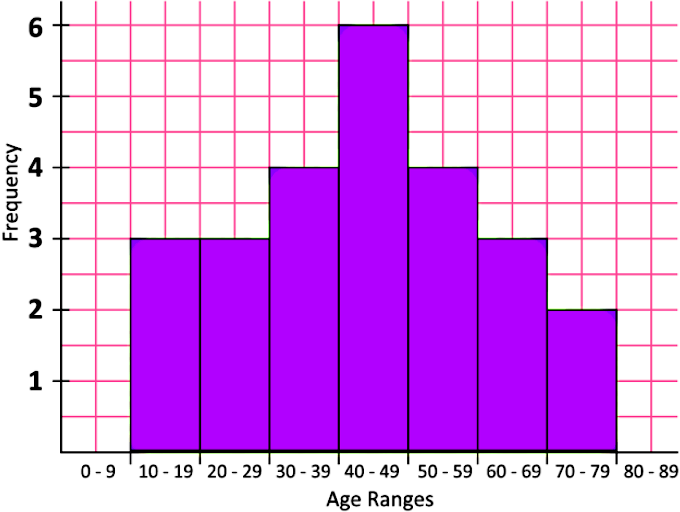Notice the central column has the highest frequency, thus, this class interval has the highest percentage of incidence from the set compared to the other classes, that is the central tendency; consequently, both the mean and median fall into this interval class too.

#### • $\quad$ Mode:

The mode of a data set is the value that appears the most within the set, the one that is repeated the most times in the set; therefore, a data set can have multiple modes or none at all.
When a data set has all different values, it means there is not a mode present; however, if there are a few values that repeat themselves equally, and are the ones that can be found the most, the data set will contain as many modes as the values that equally repeat the most.

#### ‣ $\quad$ How to find mode:

Since the mode definition says is the number that shows up the most within a set of numbers, let us take the data set coming from example 1 again:

16 $\;$18$\;$ 18 $\;$ 21$\;$ 27$\;$ 29$\;$ 30$\;$ 33$\;$ 34$\;$ 39 $\;$ 41 $\;$ 44 $\;$ 44 $\;$ 45 $\;$ 45 $\;$ 48$\;$ 54$\;$ 55$\;$ 57$\;$ 59$\;$ 61 $\;$ 63$\;$ 66 $\;$ 70 $\;$ 72

What is the mode for this data set? The the numbers 18, 44 and 45 are all modes of the data set, since they are the ones that show up the most and they all appear the same amount of times; hence, the data set from example 1 is trimodal (has three modes).

#### • $\quad$ Range:

Now that you know what is the mean, median and mode one last important value to know from a data set is its range, so let us go about the range definition:
The range of a data set is the difference between the biggest and the smallest value in the set. This is actually very intuitive, since the word range itself tells us it must be about the extent of the data set, the scope that it covers.

#### ‣ $\quad$ How to find the Range:

To find the range we just perform the subtraction between the highest and the lowest values in the set:

range = highest value - lowest value
range = 72 -16=56

Now that you have obtained the mean, median, mode and range of the data provided in example one, let us take a look at the frequency distribution graphic representations of the data set and then see where our mean, median, mode and range values fall: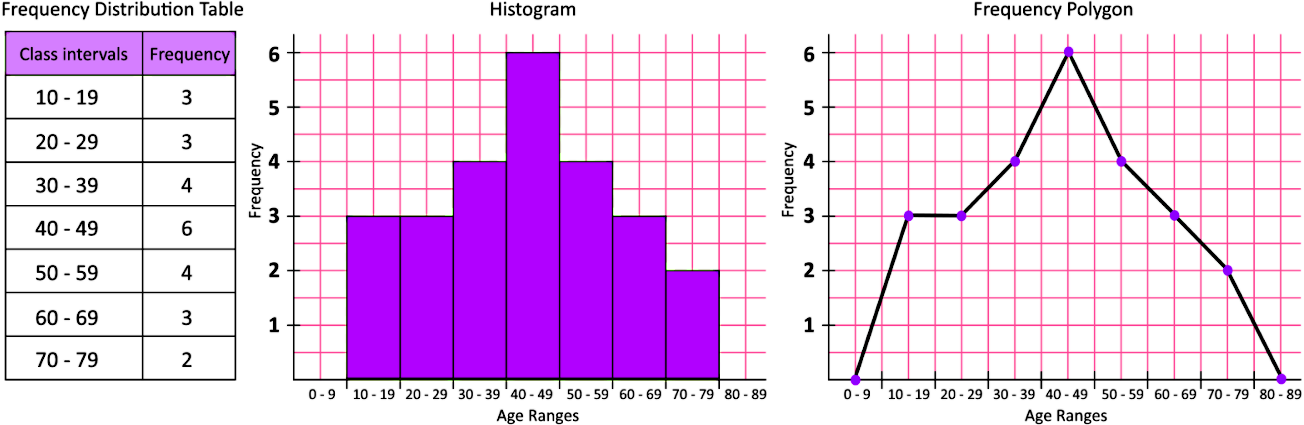#### Example 2

Determine the mean of each set of data:
1. {8, 5, 2, 12, 3}
2. {7, 5, 3, 8, 3, 4}

For the data set in part a) we compute the mean using equation 1:

$\overline{X} = \frac{8\, +\, 5\, +\, 2\, +\, 12\, +\, 3}{5} = \frac{30}{5} = 6$

For the data set in part b), we compute the mean:

$\overline{X} = \frac{7\, +\, 5\, +\, 3\, +\, 8\, +\, 3 \, +4 }{6} = \frac{30}{6} = 5$

#### Example 3

Determine the median of each set of data:
1. {8, 5, 2, 12, 3}
2. {7, 5, 3, 8, 3, 4}

For the data set in part a), we start by ordering the values from lowest to highest:
{8, 5, 2, 12, 3} = {2, 3, 5, 8, 12}

Then the value in the middle of the set is: 5, and so, median = 5

For the data set in part b), once more, we start by ordering the values from lowest to highest:
{7, 5, 3, 8, 3, 4} = {3, 3, 4, 5, 7, 8}

If this data set had an odd number of data values, the median would have been very easy to find. It would have just been the number in the middle. However, since we have an even number of data values, well take the two middle numbers and divide it by two to get the average between them. This gives us: (4+5)/2, which produces a median of 4.5.

#### Example 4

The heights (in cm) of students in a class are: {156, 152, 148, 159, 150}
1. Determine the mean and the median.
2. A new student with a height of 255 cm joins the class. How does the new data value (outlier)affect the mean and the median?

To answer part a) of our example problem we calculate the mean of this data set as follows:

$\overline{X} = \frac{156\, +\, 152\, +\, 148\, +\, 159\, +\, 150}{5} = \frac{765}{5} = 153$

Now what is the median? Easy! We order the data set from lowest to highest and pick the middle number:
{156, 152, 148, 159, 150} = {148, 150, 152, 156, 159}

And so the median is equal to 152.

For part b) of this problem we will be investigating the impact of outliers on the mean and median. Outliers are values which are very far away from the rest of the data values in the set, therefore, they can complicate the detection of the central tendency of the data set; thus, affecting the value of the mean too.

The added value to the set of 255 is an outlier because is proportionally very distant from the rest of the data set values when comparing the distances between them. The first five data values went from 148 to 159, with a range of 11; however, if we take into consideration the sixth added value of 255 the range of the data set goes up to 107! Let us now use this new data set then {148, 150, 152, 156, 159, 255} to define mean and median for this case:

For the mean:

$\overline{X} = \frac{156\,+\,152\,+\,148\,+\,159\,+\,150\,+\,255} {6} = \frac{1020}{6} = 170$

Now we find the median:
Given that now the data set contains an even amount of values, we pick the two center values and average them in order to find the median:

{148, 150, 152, 156, 159, 255} $\,$$\, \frac{152\,+\,156} {2} = \frac{308}{2} = 154$

Notice how the mean was greatly affected by the outliers presence in the data.

#### Example 5

Determine the mode of each set of data:

1. {8, 5, 2, 12, 5}

There is no mode on this set because none of the values appears more than once.

2. {6, 6, 6, 7, 7, 8, 8, 8, 9}
The values 6 and 8 both appear the most on this set, therefore this set has two modes and is called a bimodal set.

3. {1, 2, 3, 4}
Once more, there are no modes on this set because none of the values is repeated.

#### Example 6

Determine the mean for the population of scores in the following frequency table.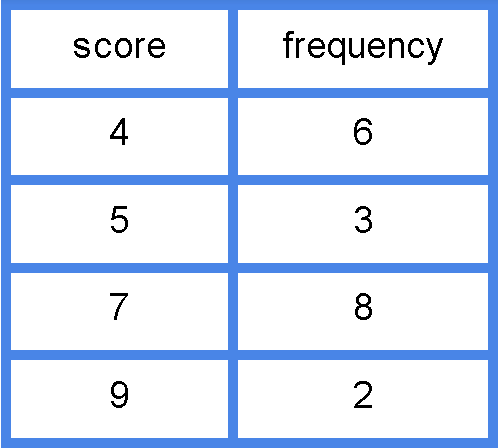From this table we obtain that the data set is: 4, 4, 4, 4, 4, 4, 5, 5, 5, 7, 7, 7, 7, 7, 7, 7, 7, 9, 9.
Therefore, the mean is:

$\overline{X} = \frac{4+4+4+4+4+4+5+5+5+7+7+7+7+7+7+7+7+9+9} {19} = \frac{113}{19} = 5.95$

So what is the median in this case? Median = 7
What is the mode? The mode is equal to the median for this case since the score with highest frequency is 7, and so, mode = 7 = median.
And finally, what is the range? Range = 9 - 4 = 5.

***

To finalize this lesson we recommend you to visit the next article on the mean, median, mode and range. And for special cases, we suggest you to take a look into this lesson explaining the relationships between mean, median and mode in special distributions.

So this is it for this lesson, see you in the next one!
3 ways for describing the "center" of a data set:

1. mean ($\overline{x}$ or $\mu$): arithmetic average of a data set

$\overline{x}= \frac{x_1+x_2...+x_n}{n}$
$x$: data value
$n$: # of items in a data set

2. median: the "middle" of a sorted list of data values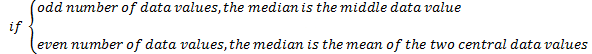3. mode: the data value that occurs most often in a data set

$\cdot$ The median is not affected by outliers, but the mean is.

$\cdot$ weighted mean($\overline{x}_{weighted}$): arithmetic average where some data values contribute more than others

$\overline{x}_{weighted}=$ $\frac{\sum_{{i=1}}^{n}x_i \cdot w_i}{\sum_{{i=1}}^{n}w_i}$